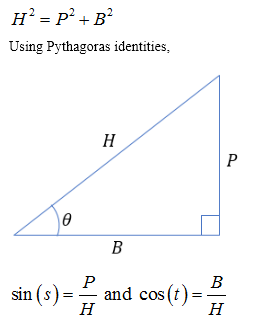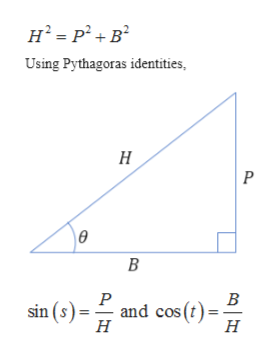# Find the exact value of the expression using the provided information.Find sin(s + t) given that cos s = -12/13 , with s in quadrant II, and  with sin t =8/17 in quadrant II

Question
3 views

Find the exact value of the expression using the provided information.

Find sin(s + t) given that cos s = -12/13 , with s in quadrant II, and  with sin t =8/17 in quadrant II

check_circle

Step 1

Given,

Step 2

Pythagoras theorem,help_outlineImage TranscriptioncloseUsing Pythagoras identities, н P ө В Р В and cos (t) sin (s) Н н fullscreen
Step 3

Formula need...

### Want to see the full answer?

See Solution

#### Want to see this answer and more?

Solutions are written by subject experts who are available 24/7. Questions are typically answered within 1 hour.*

See Solution
*Response times may vary by subject and question.
Tagged in